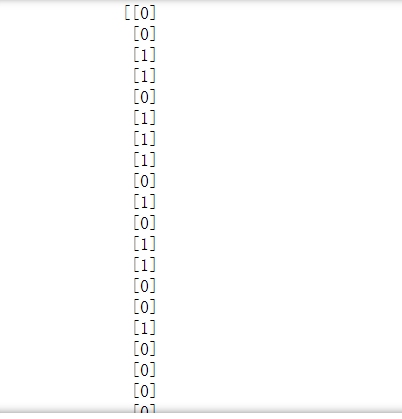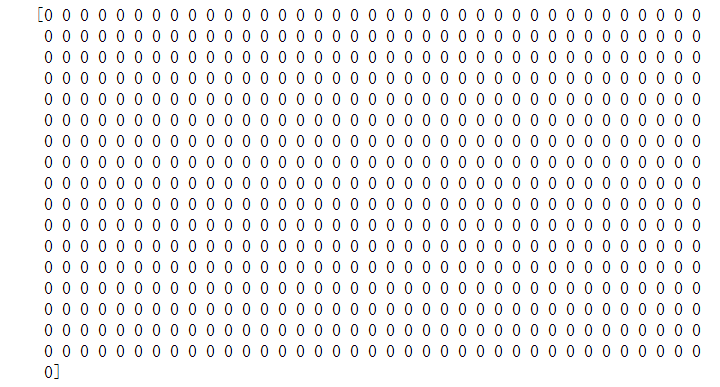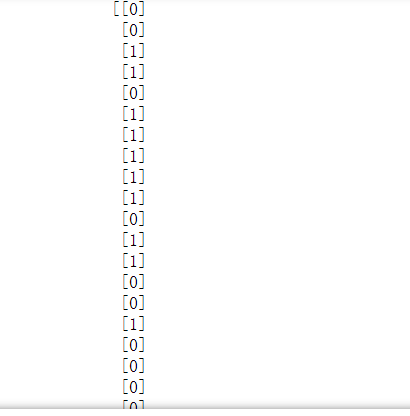# 关于新版tensorflow 'Sequential' object has no attribute 'predict_classes'的问题

``````y_train_predict = mlp.predict_classes(X_train)
print(y_train_predict)

````````````y_train_predict = mlp.predict(X_train)
y_train_predict = np.argmax(y_train_predict, axis=1)
print(y_train_predict)

``````• 写回答

#### 2条回答默认 最新

•吉吉丶丶 2022-03-30 23:52
关注

我仔细思考了一下终于解决啦！
我的数据为sigmoid函数映射到0和1之间分布的点数，我想把他以大于0.5和小于0.5分别预测为1和0，输出的结果为0 1分布，但是新版tensorflow没有predict_classes属性，所以我做了如下操作

``````y_train_predict = mlp.predict(X_train)
a = np.ones(630)
b = a/2
c = np.insert(y_train_predict,0,b,axis=1)
y_train_predict = np.argmax(c,axis=1)
y_train_predict = y_train_predict.reshape(630,1)
print(y_train_predict)
``````

生成一列0.5加入到第0列，再用np.argmax按行索引判断最大值的位置，如果0.5是最大的就返回0，如果0.5是最小的就返回1
再转化为二维数组格式，输出结果为和之前的predict_class结果基本一样

本回答被题主选为最佳回答 , 对您是否有帮助呢?
评论

#### 悬赏问题

• ¥15 java连接sqlserver有问题
• ¥15 yolov8 如何调cfg参数
• ¥15 这个四人抢答器代码哪儿有问题？仿真程序怎么写？
• ¥15 burpsuite密码爆破
• ¥15 关于#ubuntu#的问题，如何解决？（相关搜索：移动硬盘）
• ¥15 scikit安装之后import不了
• ¥15 Ros2编译一个使用opencv的c++节点的时候，报了这个错误，请问怎么解决啊
• ¥15 人脸识别相关算法 YOLO，AI等
• ¥15 spark问题方便加V17675797079
• ¥15 Python代码不打印的原因